In :
import numpy as np
import pandas as pd
from math import pi, floor
import hsluv
from matplotlib import pyplot as plt
plt.rcParams['figure.figsize'] = [14, 7]


## Measurement data¶

Measured data are layed out in one file per density, and each file contains a lines with two values, namely the number of pixels of the grain of interest that are visible, and the number of background pixels that are visible (ie pixels where the closest grain is further than Z_max). A file repeats sample_count times the same measures, so that it gives data point in the following order:

sample=1, Z=0
sample=1, Z=0.01 Z_max
...
sample=1, Z=Z_max
sample=2, Z=0
sample=2, Z=0.01 Z_max
...
sample=2, Z=Z_max
...
In :
# PARAMETERS
# template of experiment's result file, %x replaced by density
# densities for which the experiments have been run, as an inversed proportion of the max stack density
densities = np.arange(1.0, 2.075, 0.025)
# depths for which the experiments have been run
zs = np.linspace(0.00, 0.1, 100)
# radius of a grain in the experiments (use to show plots in percentage of radius)

In :
# infer sample count from the content of the first file
density_frames = densities.shape
z_frames = zs.shape
df = pd.read_csv(file_tpl % densities, sep=';')
sample_count = floor(df.values.shape / z_frames)

In :
# Load data into an array parametrized by (density, depth, sample)
data = np.zeros((density_frames, z_frames, sample_count, 2))
for i, x in enumerate(densities):
df = pd.read_csv(file_tpl % x, sep=';')
data[i,:,:,:] = df.values[:z_frames * sample_count,1:].reshape(sample_count, z_frames, 2).transpose(1, 0, 2)


## Sanity checks¶

In :
max_black = 2710 # maximum number of black pixels (pixels of the grain of interest) given its size
for i in np.unique(np.argwhere(data[:,:,:,0] > max_black)[:,0]):
print("Error with curve #{} (density={}): improbable number of black pixels".format(i, densities[i]))
continue


## Plots¶

In :
def color(t):
return hsluv.hsluv_to_rgb([
340 * (1 - t) + 250 * t,
100 * (1 - pow(t,.5)) + 60 * pow(t,.5),
50 * (1 - t) + 50 * t,
])

fig, ax = plt.subplots()
ax.set_xlabel('depth z')
ax.set_ylabel('Fraction of visibility')
for i, d in enumerate(densities[:-2]):
if i % 2 != 0: continue
curve_data = data[i,:,:,0].mean(axis=1) / max_black
ax.plot(zs / radius, curve_data, label="d=%.03f"%(1./d), color=color(i/(len(densities)-1)))
ax.legend(loc='best')
ax.set_title("Mean visibility of a grain at depth z in a sand tract\nwhose density is d times the maximum stacking density without grain overlap.\nAveraged over 1000 samples, with grains of radius 1.")

Out:
Text(0.5, 1.0, 'Mean visibility of a grain at depth z in a sand tract\nwhose density is d times the maximum stacking density without grain overlap.\nAveraged over 1000 samples, with grains of radius 1.')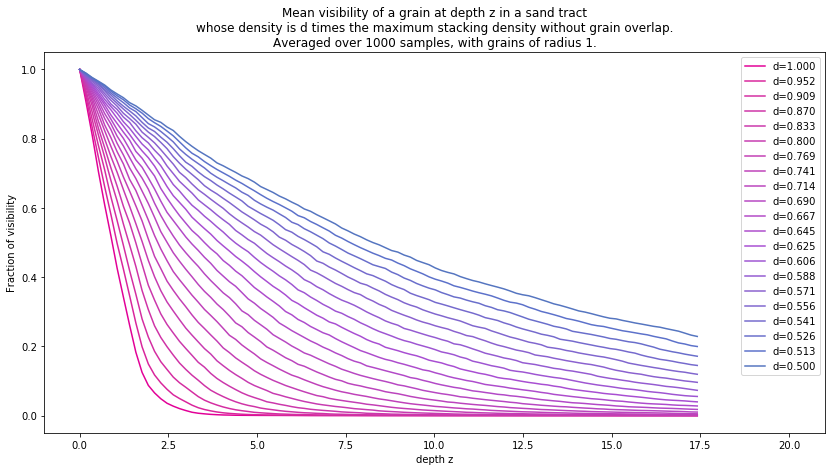In :
def plot_stddev(i):
fig, ax = plt.subplots()
ax.set_xlabel('depth z')
ax.set_ylabel('Fraction of visibility')

mean_curve = data[i,:,:,0].mean(axis=1) / max_black
ax.plot(zs / radius, mean_curve, label="mean", color=color(0))

stddev_data = data[i,:,:,0].std(axis=1) / max_black
ax.plot(zs / radius, np.minimum(1, mean_curve + stddev_data), label="mean+stddev", color=color(1))
ax.plot(zs / radius, np.maximum(0, mean_curve - stddev_data), label="mean-stddev", color=color(1))

ax.legend(loc='best')
ax.set_title("Mean visibility of a grain and its standard deviation for d=%.03f" % (1./densities[i]))

In :
plot_stddev(0)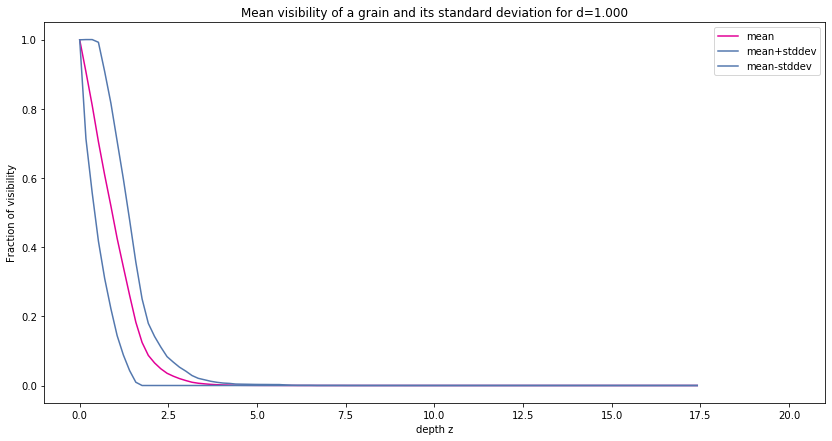In :
plot_stddev(len(densities) // 2)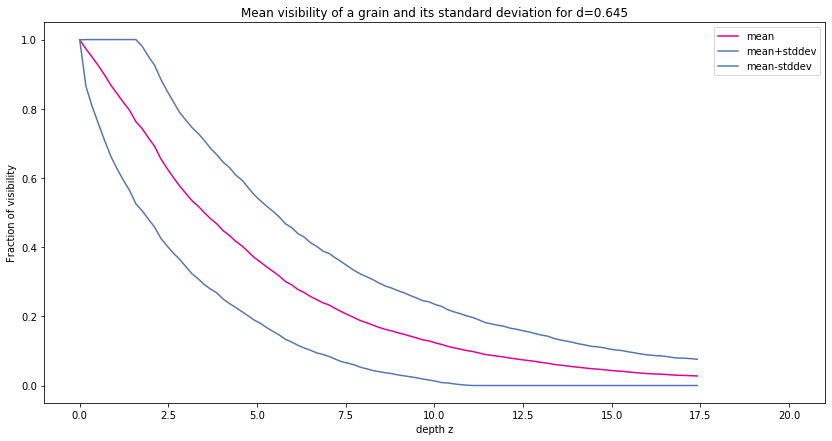In :
plot_stddev(len(densities) - 1)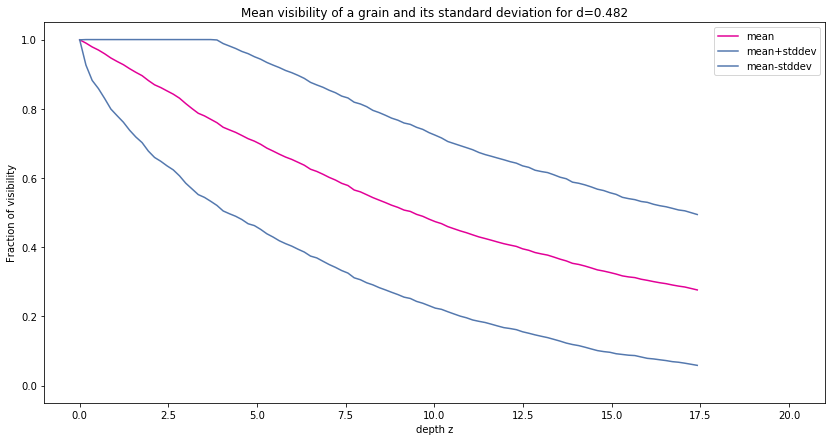## Theoretical value¶

### Jeremie's theory¶

$$\text{PVA} = \min\left(\max\left(0, 1 - \pi R^2\right)^{dz}, 1\right)$$
In :
R = 0.1
z = zs / radius * R
max_density = 0.74 / (4./3. * pi * (R*R*R))
ds = max_density / densities[:-2:2]


First issue The result should be invariant in the ratio R/z. It is indeed, unless $1-\pi R^2$ becomes negative.

Second issue This $1-\pi R^2$ is not homogeneous.

In :
fig, ax = plt.subplots()
ax.set_xlabel('depth z')
ax.set_ylabel('Fraction of visibility')

for i, d in enumerate(ds):
pva = np.minimum(np.power(np.maximum(0.0, 1.0 - pi * R * R), d * z), 1.0)
ax.plot(z / R, pva, label="d=%.03f"%(d/max_density), color=color(i/(len(ds)-1)))

ax.legend()
ax.set_title("Theoretical mean visibility of a grain at depth z in a sand tract of density is d*max")

Out:
Text(0.5, 1.0, 'Theoretical mean visibility of a grain at depth z in a sand tract of density is d*max')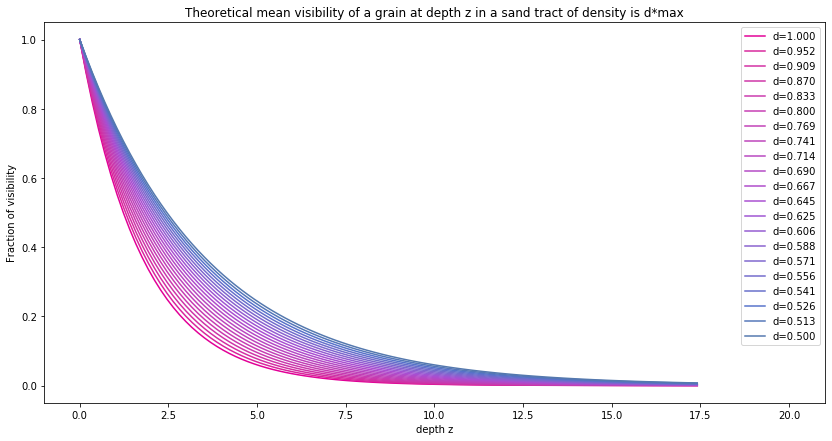Third issue The results don't match.

### Jeremie's second theory¶

$$\text{PVA} = \exp(-\pi R^2dz)$$
In :
fig, ax = plt.subplots()
ax.set_xlabel('depth z')
ax.set_ylabel('Fraction of visibility')

for i, d in enumerate(ds):
pva = np.exp(- pi * R * R * d * z)
ax.plot(z / R, pva, label="d=%.03f"%(d/max_density), color=color(i/(len(ds)-1)))

ax.legend()
ax.set_title("Theoretical mean visibility of a grain at depth z in a sand tract of density is d*max")

Out:
Text(0.5, 1.0, 'Theoretical mean visibility of a grain at depth z in a sand tract of density is d*max')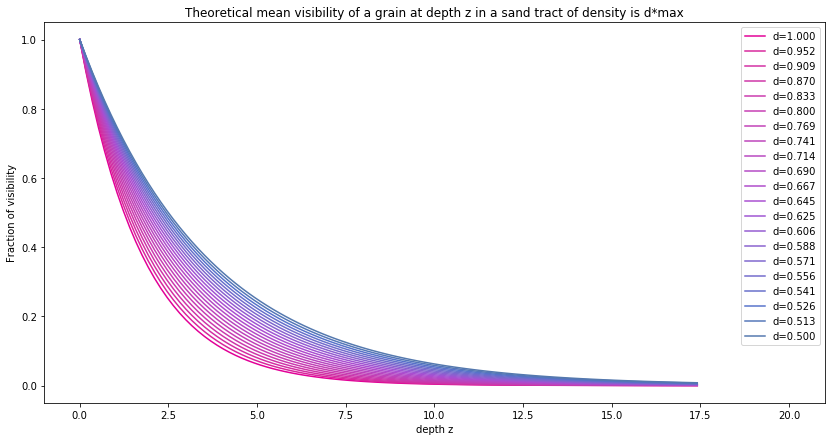## Model fitting¶

### Polynomial fitting¶

In :
x = zs / radius
degrees = np.arange(1, 10, 1, dtype=np.int32)
res = np.zeros((len(degrees), len(densities)))
for j, deg in enumerate(degrees):
for i, d in enumerate(densities):
y = data[i,:,:,0].mean(axis=1) / max_black
(p, residuals, rank, singular_values, rcond) = np.polyfit(x, y, deg, full=True)
res[j, i] = residuals

fig, ax = plt.subplots()
ax.set_xlabel('density')
ax.set_ylabel('residuals')

for j, deg in enumerate(degrees):
pva = np.exp(- pi * R * R * d * z)
ax.plot(1./densities, res[j], label="degree=%d" % deg)

ax.legend()
ax.set_title("Polynomial fitting residuals")

Out:
Text(0.5, 1.0, 'Polynomial fitting residuals')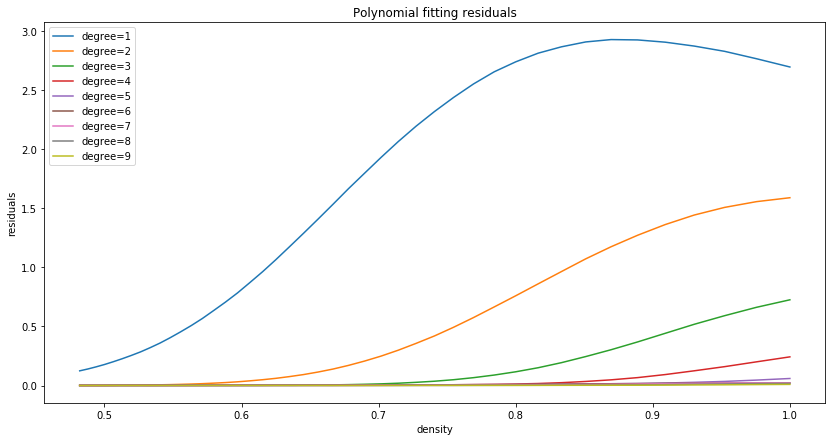Clearly it is not fitting.

In :
# sanity check about the use of np.polyfit
p = [1.0, 0.2, -1.6, -1.3, -1.2]
curve_data = eval_polynomial(x, p)

(p2, residuals, rank, singular_values, rcond) = np.polyfit(x, curve_data, 5, full=True)

fig, ax = plt.subplots()
ax.plot(x, curve_data)
ax.plot(x, eval_polynomial(x, p2))
residuals

Out:
array([1.98199437e-21])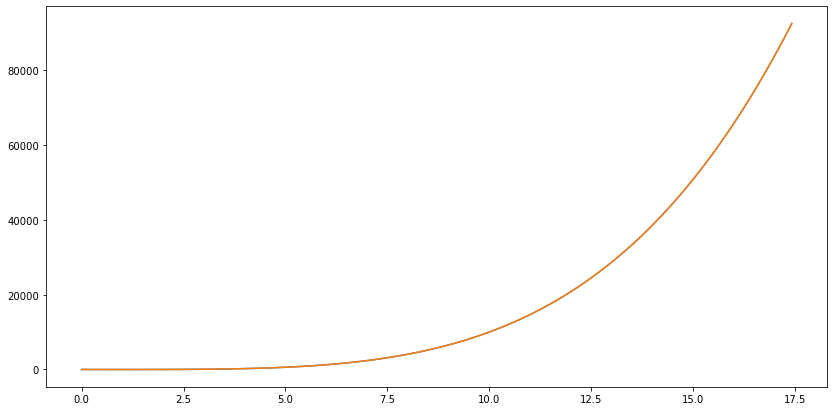Ok.

### Exponential polynomial¶

Model is $\exp(P(z))$ where $P$ is a polynomial function.

In :
x = zs / radius
degrees = np.arange(1, 10, 1, dtype=np.int32)
res = np.zeros((len(degrees), len(densities)))
for j, deg in enumerate(degrees):
for i, d in enumerate(densities):
y = np.log(data[i,:,:,0].mean(axis=1) / max_black)
(p, residuals, rank, singular_values, rcond) = np.polyfit(x, y, deg, full=True)
res[j, i] = residuals

fig, ax = plt.subplots()
ax.set_xlabel('density')
ax.set_ylabel('residuals')

for j, deg in enumerate(degrees):
pva = np.exp(- pi * R * R * d * z)
ax.plot(1./densities, res[j], label="degree=%d" % deg)

ax.legend()
ax.set_title("Exponential polynomial fitting residuals")

Out:
Text(0.5, 1.0, 'Exponential polynomial fitting residuals')